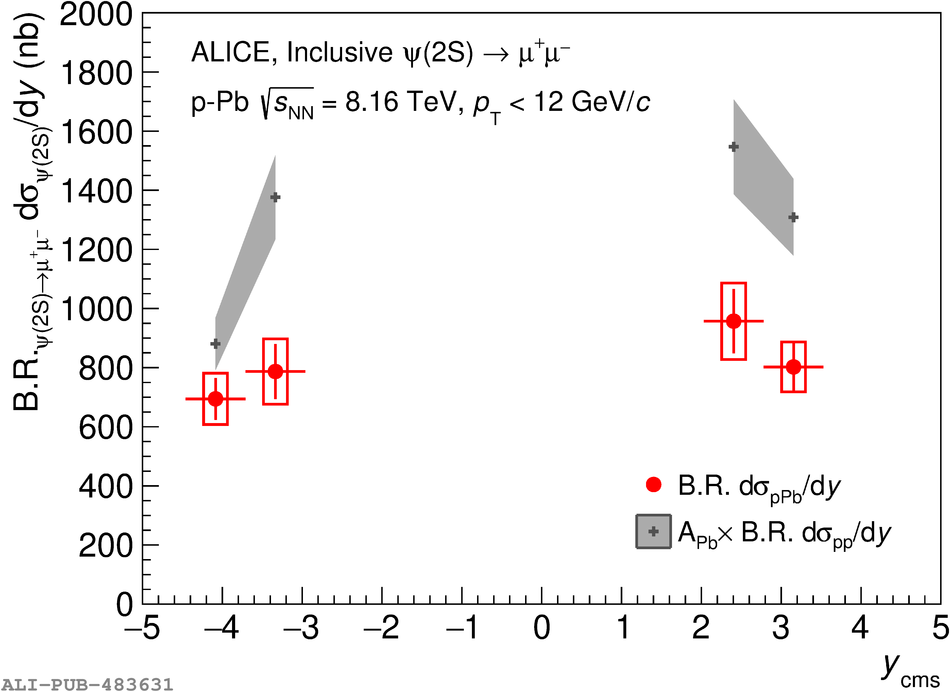# Figure 2

 The differential cross section times branching ratio ${\rm B.R.}_{\psi(2{\rm S})\rightarrow\mu^+\mu^-}{\rm d}\sigma^{\psi(2{\rm S})}/{\rm d}y$ for \$p_{\rm T}